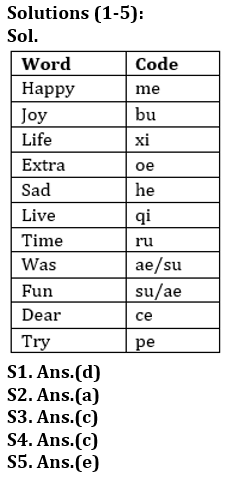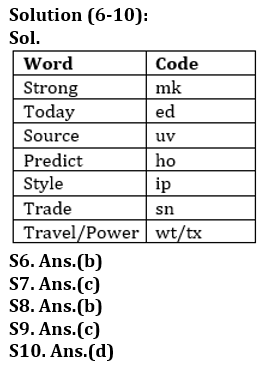Latest Banking jobs   »

# Reasoning Ability Quiz For Bank Foundation 2023-16th March

Directions (1-5): Study the following information carefully and answer the questions.
In a certain code language,
‘Happy joy life extra’ is written as ‘xi me bu oe’,
‘Sad live joy time’ is written as ‘bu ru qi he’,
‘Extra live was fun’ is written as ‘ae su qi oe’,
‘Dear sad try life’ is written as ‘pe he ce xi’,
‘Was dear fun’ is written as ‘ae ce su’.

Q1. What is the code for ‘life’?
(a) me
(b) su
(c) qi
(d) xi
(e) None of these

Q2. Which of the following is the code for ‘try extra happy’?
(a) me oe pe
(b) su qi ce
(c) oe he pe
(d) me ce he
(e) None of these

Q3. What is the code for ‘time’?
(a) su
(b) bu
(c) ru
(d) Can’t be determined
(e) pe

Q4. Which of the following word is coded as ‘he’?
(a) Try
(b) Joy
(d) Happy
(e) None of these

Q5. What may be the code for ‘Live Life Fun’?
(a) qi me ru
(b) qi xi su
(c) bu xi su
(d) ae xi qi
(e) Either (b) or (d)

Directions (6-10): Study the following information carefully to answer the given questions. In a certain code language,
“Strong predict today source” is coded as “ho mk uv ed”
“Style trade Strong” is coded as “ip mk sn”
“Today trade style” is coded as “ip sn ed”
“Trade source travel power” is coded as “sn wt uv tx”

Q6. What is the code of ‘predict’?
(a) mk
(b) ho
(c) uv
(d) ed
(e) ip

Q7. What does ‘ip sn’ stands for?
(a) Today Style
(e) Can’t be determined

Q8. What may be the code of ‘trade life’?
(a) ip bh
(b) sn nj
(c) wt sn
(d) ed uv
(e) sn uv

Q9. What is the code of “predict source”?
(a) uv wt
(b) mk ed
(c) ho uv
(d) uv ec
(e) None of these

Q10. What is the code of ‘Power’?
(a) wt
(b) uv
(c) tx
(d) Either (a) or (c)
(e) ed

Directions (11-15): In these questions, relationship between different elements is show in the statements. The statements are followed by conclusions. Study the conclusions based on the given statements and select the appropriate answer:
(a) If only conclusion I follows.
(b) If only conclusion II follows.
(c) If either conclusion I or II follows
(d) If neither conclusion I nor II follows.
(e) If both conclusions I and II follow.

Q11. Statements: T<N≤P<O>R>Z=V≥I
Conclusion I: O>V II: I<P

Q12. Statements: M<P≤C >I=G>Y=D>W
Conclusion I: M>Y II: M≤Y

Q13. Statements: Z>S≥C>N =R>K<T=E>Y
Conclusion I: N=E II: Z>K

Q14. Statements: A≤P=T≤U≥L>C=K>S≥V
Conclusion I: U≥A II: V<U

Q15. Statements: T>W≤N=Z>D≥I>O≥K=J
Conclusion I: J<O II: N≥I

SolutionsSolutions (11-15):
S11. Ans.(a)
Sol. I: O>V (True) II: I<P (False)

S12. Ans.(c)
Sol. I: M>Y (False) II: M≤Y (False)

S13. Ans.(b)
Sol. I: N=E (False) II: Z>K (True)

S14. Ans.(e)
Sol. I: U≥A (True) II: V<U (True)

S15. Ans.(d)
Sol. I: J<O (False) II: N≥I (False)## FAQs

### What is the selection process of the Bank Clerk?

The selection process of the Bank Clerk is Prelims & Mains.

#### Congratulations!Union Budget 2023-24: Free PDF